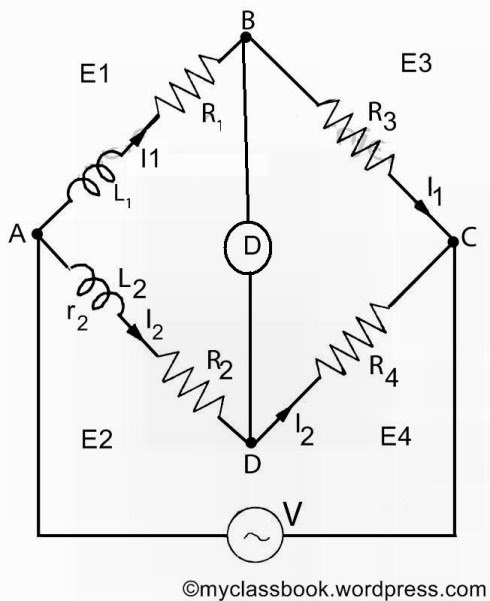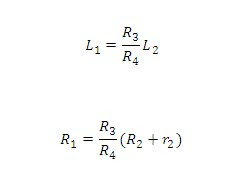# Maxwell’s inductance bridge

## Maxwell’s inductance bridge:Maxwell’s inductance bridge measures the value of given inductance by comparison with a variable standard self inductance. The circuit diagram of Maxwell’s inductance bridge is shown in figure below.Maxwells inductance bridge for measurement of inductance

Let

L1 = unknown inductance of resistance R1,

L2 = variable inductance of fixed resistance r2,

R2 = variable resistance connected in series with inductor L2,

R3, R4 = known non-inductive resistances.

At balance,Resistance R3 and R4 are normally a selection of values from 10, 100, 1000 and 10,000O. r2 is decade resistance box. In some cases, an additional known resistance may have to be inserted in series with unknown coil in order to obtain balance.

Let us solve one simple problem for clear understanding of Maxwell's inductance bridge.

### Problem:

A Maxwell’s inductance comparison bridge is shown in the figure above. Arm ab consists of a coil with inductance L1 and resistance r1 in series with a non-inductive resistance R. arm bc and ad are each a non-inductive resistance of 100O. Arm ad consists of standard variable inductor L of resistance 32.7O. Balance is obtained when L2 = 47.8mH and R = 1.36O. Find the resistance and inductance of the coil in arm ab.

### Solution:

At balance [(R1+r1)+jwL1]*100 = (r2+jwL2)*100

Equating the real and imaginary terms

R1+r1 = r2 and L2=L1

Therefore, resistance of coil:

r1 = r2 – R1 = 32.7 – 1.36 = 31.34O.

Inductance of coil:

L1 = L2 = 47.8mH.

tags: maxwell's inductance bridge ppt pdf maxwell's bridge theory anderson bridge for the measurement of inductance. maxwell bridge experiment applications uses and disadvantages and advantages of maxwell inductance bridge.

1.2.3.